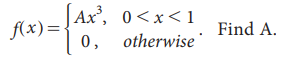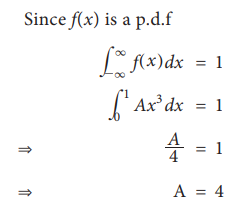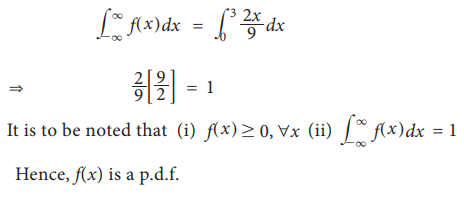Home | | Statistics 11th std | Probability Density Function

# Probability Density Function

The probability function associated with continuous random variable is called probability density function.

## Probability mass function and probability density function

A probability function is associated with each value of the random variable. This function is used to compute probabilities for events associated with the random variables. The probability function defined for a discrete random variable is called probability mass function. The probability function associated with continuous random variable is called probability density function.Probability Density Function

When the random variable is continuous in the co-domain it is spread over it. A function f (x) is defined on real line and satisfying the following conditions :is called the probability density function(p.d.f) of X.### Example 9.10

A  continuous  random  variable  X has  probability density function given by### Solution:### Example 9.11

Verify whether the following function is a probability density function### Solution:Study Material, Lecturing Notes, Assignment, Reference, Wiki description explanation, brief detail
11th Statistics : Chapter 9 : Random Variables and Mathematical Expectation : Probability Density Function |